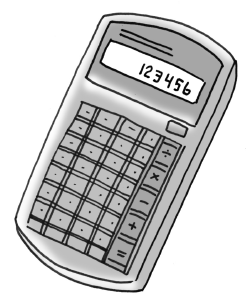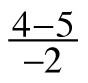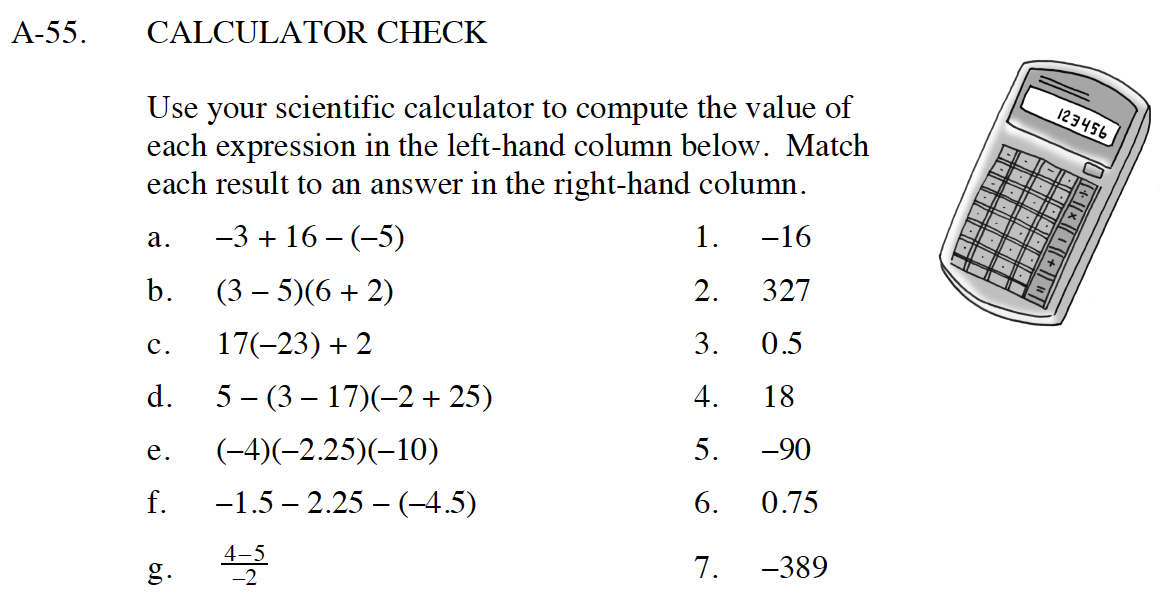Home > CC4 > Chapter A > Lesson A.1.5 > ProblemA-55

A-55.
1.A-55. CALCULATOR CHECK

2. Use your scientific calculator to compute the value of each expression in the left-hand column below. Match each result to an answer in the right-hand column. Homework Help ✎

 a. −3 + 16 − (−5) 1. −16 b. (3 − 5)(6 + 2) 2. 327 c. 17(−23) + 2 3. 0.5 d. 5 − (3 − 17)(−2 + 25) 4. 18 e. (−4)(−2.25)(−10) 5. −90 f. −1.5 − 2.25 − (−4.5) 6. 0.75 g.7. −389Remember to be cautious when entering negative signs.
A few matches have been made above, so that you can check some of your work.